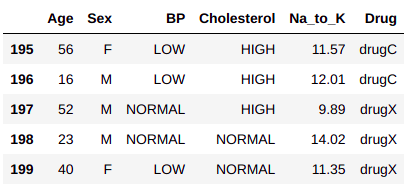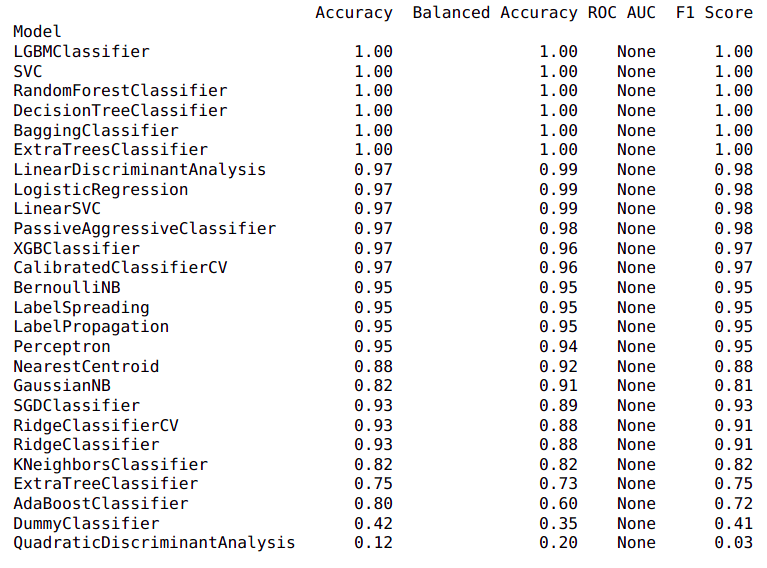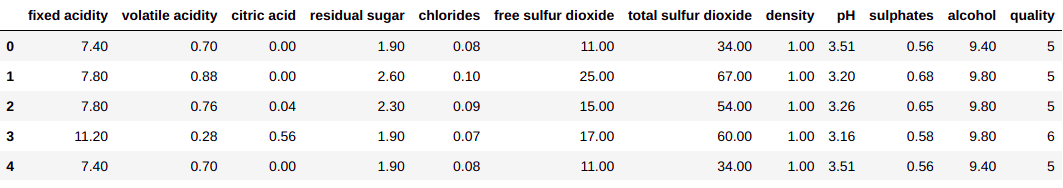As in the earlier blog “text classification using machine learning”, we saw a few drawbacks on how difficult it is to select the best ML models and time-consuming for tuning different model parameters to achieve better accuracy.  To overcome this problem we will discuss here an awesome python library “Lazy Predict”. This module helps us find the best model for classification and regression based on our data.

It provides a Lazy Classifier for classification problems and Lazy Regression for regression problems.

• Note: Lazy Predict takes high computational power and it was a little time-consuming for me to run high dimensional data with multiple features.

Let us see how it works:

First, install this library in your local system

pip  install lazypredict

### Dataset

Here we are not concentrating more on the dataset or its feature extraction and transformation steps, as it has been shown in the previous blog on “text classification using machine learning”.

To demonstrate lazy predict classification and regression problems we are using “Drug type” and “Wine quality” data both taken from kaggle.com

### Code

#### Importing required libraries

import lazypredict

import pandas as pd

from sklearn.model_selection import train_test_split

from lazypredict.Supervised import LazyClassifier, LazyRegressor

#### Importing data and LazyClassifier model fittingX = classificationData..drop(columns=”Drug”)

y = classificationData.[“Drug”]

# Splitting our data into a train and test set

X_train, X_test, y_train, y_test = train_test_split(X, y,

test_size=0.2,

random_state=42)

classifiers = LazyClassifier(ignore_warnings=True, custom_metric=None)

models,predictions = classifiers.fit(X_train, X_test, y_train, y_test)

print(models)Here the model returns two values, different model names with its prediction accuracy.

#### Importing data and LazyRegressor model fittingX = regressionData.drop(columns=”quality”)

y = regressionData[“quality”]

# Splitting our data into a train and test set

X_train, X_test, y_train, y_test = train_test_split(X, y,

test_size=0.2, random_state = 42)

regressors = LazyRegressor(ignore_warnings=True, custom_metric=None)

models, predictions = regressors.fit(X_train, X_test, y_train, y_test)

print(models)### Conclusion

Here, when we use the “Lazy Predict” library, different models are fitted on our data, and model results provide us with accuracy metrics for the given data. Observing the result we can then select the top 5 base models based on the best accuracy.

Later we can tune the parameters of those top models and get better accuracy.

As this library runs many different models at once it takes a lot of computational power. If you have low computational power I would suggest you use Google Colab.